Courses

# Classification CLAT Notes | EduRev

## CLAT : Classification CLAT Notes | EduRev

The document Classification CLAT Notes | EduRev is a part of the CLAT Course Logical Reasoning for CLAT.
All you need of CLAT at this link: CLAT

Chapter - 5

Classification

In these types of questions, the students are required to find out the ‘odd one’ out. The candidate is given five terms and out of those terms, one does not match with the others which is called ‘odd one’ in the group. The students are required to identify it on the basis of common trait observed in the other four terms.

TYPE – I

In this type of questions, a groups of letters is provided, in which you are required to select that group of letters as your answer, in which letters do not follow the same pattern as that of the other groups of letters. The letters in a group are arranged on the basis of relative distance between the two letters as in the English alphabet; In other words, certain number of letters are skipped between the two successive letters in the given group of letters. It will become more clear by comprehending the examples given below:

Ex. 1.  Four of the following five are alike on the basis of their position in English alphabet. Which is the one that does not belong to the group?

(1) WZT
(2) KNH
(3) RUQ
(4) DGA

Solution: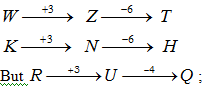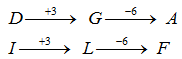But ;  So RUQ is an odd one, So answer is   (3)

Ex.  2. Four of the following five are alike in a certain way with regard to their position in English alphabet. Which one does not belong to that group?

(1) IJK
(2) ABC
(3) OPQ
(4) VWX

Answer:    (4)

All the options start with vowel like I, A, O, E but only 4th option start with V i.e. a consonant, so answer is (4)

Ex. 3.   Four of the following five are alike in respect to their position in the English alphabet and hence form a group. Which one does not belong to that group?

(1) CFI
(2) UXA
(3) RUX
(4) SVZ

Solution: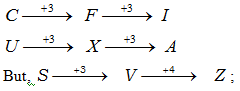But,;                                   So SVZ is odd one, so answer is (4)

Ex.  4. Four of the following five are alike in a certain way and so form a group with respect to their position in English alphabet. Which is the one that does not belong to the group?

(1) KNR
(2) ADI
(3) CFJ
(4) HKO

Solution: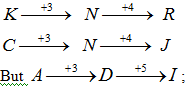But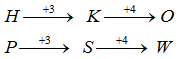;    So ADI is odd one, so answer is (2)

Ex. 5. Four of the following five are alike in a certain way and hence form a group. Which is the one that does not belong the group?

(1) WHITE-IWEHT
(2) BLACK-ABKLC
(3) TYPES-PTSYE
(4) ABOUT-OATUB

Solution:  (4), In all the options first letter goes to 4th position in new arrangement second letter goes to 4th position in the new arrangement except in 4th option because in this second letter goes to fifth position

TYPE – II

In this type, we have included such questions which contain some items which have certain common property and these type of questions are generally based on general knowledge. Here, you are required to find out the item which can not be grouped with other items for the lack of common property. It should be noted that the same item can be grouped differently in the different context. Therefore, the process of grouping depends much on the nature of the given items. Consider the following examples.

Directions (Q. 6-25):  Four of the following five are alike in a certain way and so form a group. Which is the one that does not belong to that group?

Ex.  6.
(1) Earth
(2) Saturn
(3) Moon
(4) Sun

Ex.  7.
(1) Earth
(2) Saturn
(3) Moon
(4) Mars

Ex.  8.
(1) Horse
(2) Donkey
(3) Cow
(4) Dog

Ex.  9.
(1) June
(2) Year
(3) Week
(4) Month

Ex.  10.
(1) Dog
(2) Cat
(3) Horse
(4) Zebra

Ex.  11.
(1) Apple
(2) Orange
(3) Papaya
(4) Rose

Ex.  12.
(1) Tiger
(2) Cow
(3) Bitch
(4) Mare

Ex.  13.
(1) Pear
(2) Mango
(3) Orange
(4) Radish

Ex.  14.
(1) Jumping
(2) Running
(3) Walking
(4) Exercising

Ex.  15.
(1) Novel
(2) Poetry
(3) Drama
(4) Literature

Ex. 16
(1) Gums
(2) Arm
(3) Hand
(4) Tears

Ex. 17
(1) Follow
(2) Chase
(3) Pursue
(4) Investigate

Ex. 18
(1) Lion
(2) Cat
(3) Donkey
(4) Animal

Ex. 19
(1) Cube
(2) Square
(3) Rectangle
(4) Parallelogram

Ex. 20
(1) Anxiety
(2) Worry
(3) Inhibition
(4) Curiosity

Ex. 21
1) Volume
2) Size
3) Large
4) Shape

Ex. 22
1) August
2) December
3) July
4) May

Ex. 23
1) Jackal
2) Cheetah
3) Tiger
4) dog

Ex. 24
1) Cheese
2) Butter
3) Milk
4) Curd

Ex. 25
1) Sultry
2) Hot
3) Humid
4) Cool

ANSWERS WITH EXPLANATION

6.(4)Sun is the odd one because all others are revolving heavenly bodies while the position of Sun is stationary. Earth, Saturn and Jupiter are planets, which revolve around the Sun whereas Moon is a satellite which revolves round the planet Earth.

7. (3)  Moon is the odd one because all others are  planets and Moon is a satellite.
8. (4) Except dog, all other animals are herbivores.
9. (1)  June is a name of the month.
10. (4) Zebra is a wild animal.
11. (4) Except rose, all others are fruits. Rose is a flower.
12. (1) Except Tiger, all others are females.
13. (4) Except Radish all others are fruit.
14. (4) All others are different modes of exercising.
15. (4) All others are different forms of literature.
16. (4) All other are parts of human body.
17. (4) All other are synonyms.
18. (4) All other are animal.
19. (1) All other are two dimensional figures.
20. (4) All other are negative traits.
21. (3) ‘Large’ is an adjective whereas others are noun.
22. (4) Except it others are either followed or preceded by a month of 31 days.
23. (4) Others are wild animals.
24. (3) Others are the products made from milk.
25. (4) Except it others are synonymous to each others.

TYPE – III

In this type of questions, the principle of Numeral Analogy has been applied. Out of the given numbers, one does not show similar property and that number is selected as the answer. The grouping of numbers can be done on the basis of following properties of number:

(i)  Prime Numbers
(ii) Rational Numbers
(iii) Irrational Numbers
(iv) Squares and Cubes

Now consider the following examples:

Directions (Q. 26-35):  Four of the following five are alike in a certain way and so form a group. Which is the one that does not belong to that group?

Ex. 26.
(1) 21
(2) 51
(3) 63
(4) 23

Ex. 27.
(1) 13
(2) 17
(3) 19
(4) 27

Ex. 28.
(1) 117
(2) 74
(3) 153
(4) 108

Ex. 29.
(1) 13
(2) 37
(3) 73
(4) 39

Ex. 30.
(1) 26
(2) 10
(3) 48
(4) 170

Ex. 31.
(1) 9
(2) 25
(3) 49
(4) 35

Ex. 32.
(1) 96
(2) 87
(3) 73
(4) 124

Ex. 33.
(1) 88

(2) 126
(3) 128
(4) 96

Ex. 34.
(1) 512
(2) 26
(3) 728
(4) 124

Ex. 35.
(1) 113
(2) 51
(3) 23
(4) 97

ANSWERS WITH EXPLANATION

26.  (4)   23 is a Prime Number. All other numbers are multiples of 3.

21 = 3 ´ 7; 51 = 3 ´ 17
63 = 3 ´ 21; 15 =  3 ´ 5

27.  (4)  Except 27, all other are Prime Numbers.

28.  (2)  Except 74, all other numbers are completely divisible by 3 or 9.

117 / 9 = 13 and 117 / 3 = 39

153 / 9 = 17 and 153 / 3 = 51

108 / 9 = 12 and 99 / 3 = 33

But, 74 / 9 = 8.22    and 74 / 3 = 24.6.

29.  (4)  Except the number 39, all other numbers are Prime Numbers.

30.  (3)  Except the number 48, all other numbers are  one more than the square of a certain number. Thus,

26 = (5)2 + 1;    10 = (3)3 + 1;

170 = (13)2 + 1;    290 = (17)2 + 1

31. (4)  All others are squares except 35.

32. (3) All others are composite numbers except 73.

33. (2) All others are divisible by 8 except 126.

34. (1)  All others are in the form x3 – 1 except 512, as it is perfect cubes of 8.

35. (2) All others are prime numbers except 51.

Offer running on EduRev: Apply code STAYHOME200 to get INR 200 off on our premium plan EduRev Infinity!

## Logical Reasoning for CLAT

29 videos|19 docs|66 tests

,

,

,

,

,

,

,

,

,

,

,

,

,

,

,

,

,

,

,

,

,

;